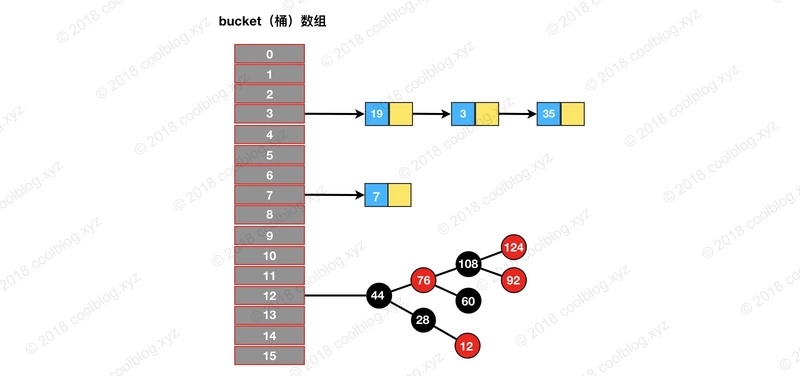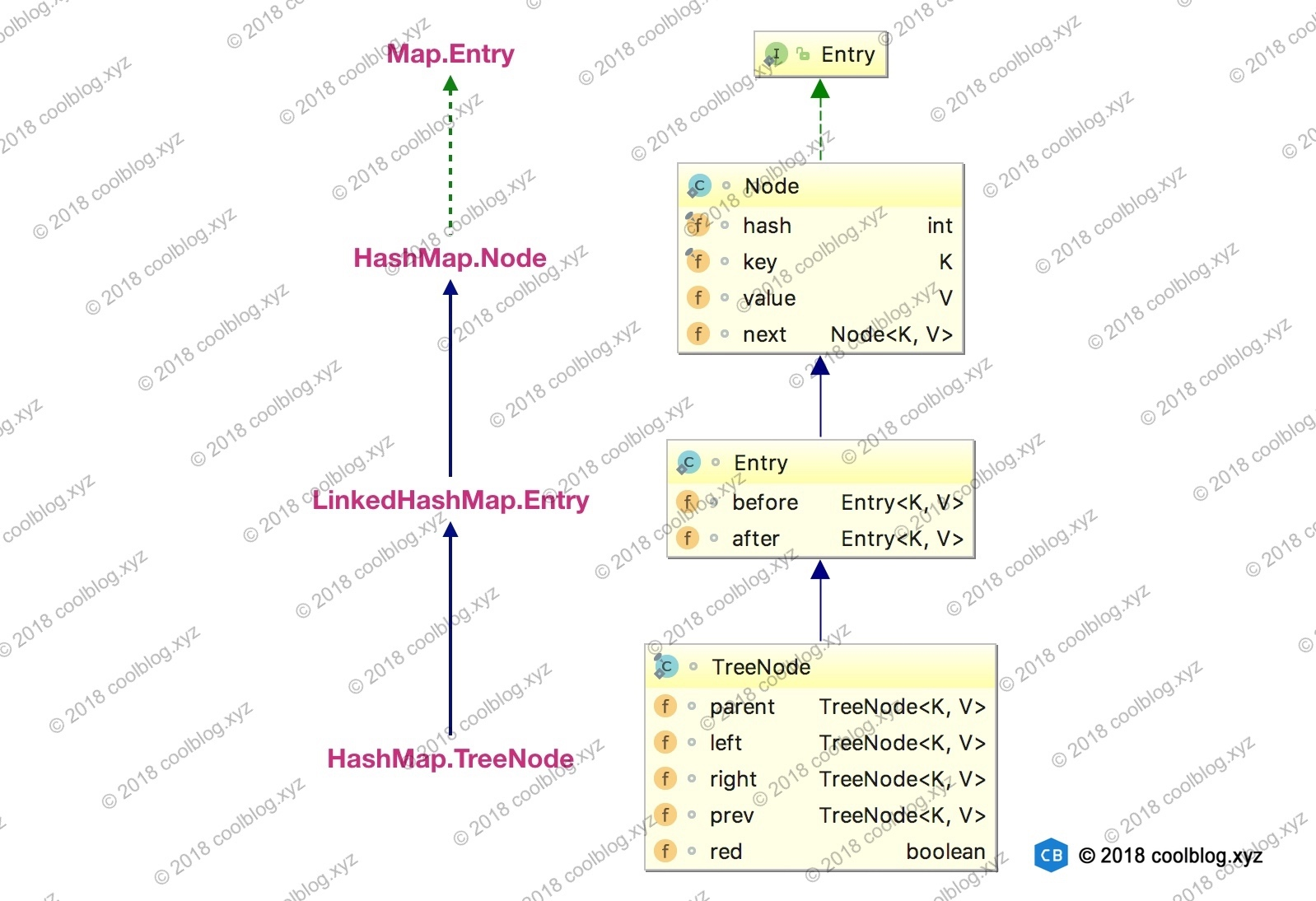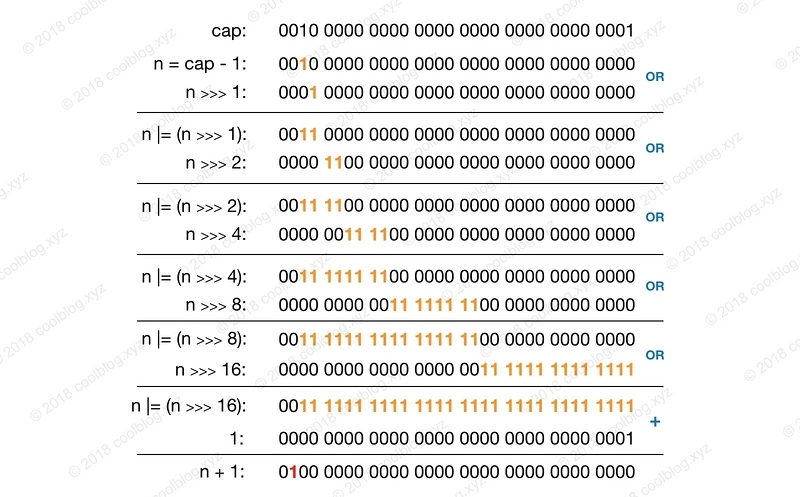# 集合系列 Map（十二）：HashMap

HashMap 是 Map 基于哈希散列算法的实现，其在 JDK1.7 中采用了数组+链表的数据结构。在 JDK1.8 中为了提高查询效率，采用了数组+链表+红黑树的数据结构。本文所有讲解均基于 JDK1.8 进行讲解。

public class HashMap<K,V> extends AbstractMap<K,V>
implements Map<K,V>, Cloneable, Serializable


## 原理

### 类成员变量

// 默认大小
static final int DEFAULT_INITIAL_CAPACITY = 1 << 4; // aka 16
// 最大大小
static final int MAXIMUM_CAPACITY = 1 << 30;
// 默认扩展因子
static final float DEFAULT_LOAD_FACTOR = 0.75f;
// 树化阈值。当超过这个阈值时，链表转成红黑树。
static final int TREEIFY_THRESHOLD = 8;
// 链化阈值。当低于这个阈值时，红黑树转成链表。
static final int UNTREEIFY_THRESHOLD = 6;
// 允许树化的最小容量。只有容量超过此值时，才允许进行树化操作。
static final int MIN_TREEIFY_CAPACITY = 64;
// 桶数组
transient Node<K,V>[] table;
// 大小
transient int size;
// 调整阈值
int threshold;
// 扩展因子
// 省略其他不重要的变量### 构造方法

HashMap 一共有 4 个构造方法。

public HashMap() {
}

public HashMap(int initialCapacity) {
}

public HashMap(int initialCapacity, float loadFactor) {
if (initialCapacity < 0)
throw new IllegalArgumentException("Illegal initial capacity: " + initialCapacity);
if (initialCapacity > MAXIMUM_CAPACITY)
initialCapacity = MAXIMUM_CAPACITY;
throw new IllegalArgumentException("Illegal load factor: " +
this.threshold = tableSizeFor(initialCapacity);
}

public HashMap(Map<? extends K, ? extends V> m) {
putMapEntries(m, false);
}


this.threshold = tableSizeFor(initialCapacity);


static final int tableSizeFor(int cap) {
int n = cap - 1;
n |= n >>> 1;
n |= n >>> 2;
n |= n >>> 4;
n |= n >>> 8;
n |= n >>> 16;
return (n < 0) ? 1 : (n >= MAXIMUM_CAPACITY) ? MAXIMUM_CAPACITY : n + 1;
}public HashMap(int initialCapacity, float loadFactor) {
if (initialCapacity < 0)
throw new IllegalArgumentException("Illegal initial capacity: " + initialCapacity);
if (initialCapacity > MAXIMUM_CAPACITY)
initialCapacity = MAXIMUM_CAPACITY;
throw new IllegalArgumentException("Illegal load factor: " +
this.threshold = tableSizeFor(initialCapacity);
}


### 核心方法

#### get

HashMap 的查找操作比较简单，首先定位键值对所在桶的位置，之后再对链表或红黑树进行查找。

public V get(Object key) {
Node<K,V> e;
return (e = getNode(hash(key), key)) == null ? null : e.value;
}

final Node<K,V> getNode(int hash, Object key) {
Node<K,V>[] tab; Node<K,V> first, e; int n; K k;
// 1.桶不为空，那么进行查找，否则直接返回 null。
if ((tab = table) != null && (n = tab.length) > 0 &&
(first = tab[(n - 1) & hash]) != null) {
// 1.1 检查要查找的是否是第一个节点
if (first.hash == hash && // always check first node
((k = first.key) == key || (key != null && key.equals(k))))
return first;
// 1.2 沿着第一个节点继续查找
if ((e = first.next) != null) {
// 1.2.1 如果是红黑树，那么调用红黑树的方法查找
if (first instanceof TreeNode)
return ((TreeNode<K,V>)first).getTreeNode(hash, key);
// 1.2.2 如果是链表，那么采用链表的方式查找
do {
if (e.hash == hash &&
((k = e.key) == key || (key != null && key.equals(k))))
return e;
} while ((e = e.next) != null);
}
}
return null;
}


first = tab[(n - 1) & hash]) != null


HashMap 中的桶数组大小总是为 2 的幂。在这个情况下，(n - 1) & hash 等价于对 length 取余。但取余的计算效率没有位运算高，所以(n - 1) & hash也是一个小的优化。

/**
* 计算键的 hash 值
*/
static final int hash(Object key) {
int h;
return (key == null) ? 0 : (h = key.hashCode()) ^ (h >>> 16);
}#### put

public V put(K key, V value) {
return putVal(hash(key), key, value, false, true);
}


final V putVal(int hash, K key, V value, boolean onlyIfAbsent,
boolean evict) {
Node<K,V>[] tab; Node<K,V> p; int n, i;
// 1. 如果未初始化，那么调用 resize() 进行初始化
if ((tab = table) == null || (n = tab.length) == 0)
n = (tab = resize()).length;
// 2. 如果桶为空，那么直接插入
if ((p = tab[i = (n - 1) & hash]) == null)
tab[i] = newNode(hash, key, value, null);
else {
Node<K,V> e; K k;
// 2.1 如果插入的元素与桶第一个元素相同，那么直接跳出
if (p.hash == hash &&
((k = p.key) == key || (key != null && key.equals(k))))
e = p;
// 2.2 如果第一个元素是红黑树节点，那么调用红黑树的插入方法
else if (p instanceof TreeNode)
e = ((TreeNode<K,V>)p).putTreeVal(this, tab, hash, key, value);
// 2.3 如果是链表节点，那么遍历到链表尾部插入。但如果中间找到了相同的节点，那么直接退出
else {
for (int binCount = 0; ; ++binCount) {
if ((e = p.next) == null) {
p.next = newNode(hash, key, value, null);
if (binCount >= TREEIFY_THRESHOLD - 1) // -1 for 1st
treeifyBin(tab, hash); //判断是否树化
break;
}
if (e.hash == hash &&
((k = e.key) == key || (key != null && key.equals(k))))
break;
p = e;
}
}
// 3. 如果插入的key已经存在，那么根据参数判断是否替代旧值
// 这里的 e 如果不为 null，那么就存储着旧值
if (e != null) { // existing mapping for key
V oldValue = e.value;
if (!onlyIfAbsent || oldValue == null)
e.value = value;
afterNodeAccess(e);
return oldValue;
}
}
++modCount;
// 4.判断是否扩容
if (++size > threshold)
resize();
afterNodeInsertion(evict);
return null;
}


1. 如果桶数组 table 为空，那么通过扩容的方式初始化 table 数组。
2. 如果插入的桶为空，那么直接插入。如果插入的桶不为空，那么判断是否与该桶第一个节点相同。如果相同，那么直接退出。否则根据节点不同类型，调用不同的插入方式。如果是红黑树节点，那么调用 putTreeVal 方法。如果是链表节点，那么直接插入链表尾部。在遍历链表的过程中，会判断节点是否存在。如果存在，则会直接跳出循环。
3. 根据条件判断 key 是否存在，如果存在则根据参数判断是否替换旧值。
4. 最后根据参数判断是否扩容。

#### remove

HashMap 的删除操作并不复杂，仅需三个步骤即可完成。第一步是定位桶位置，第二步遍历链表并找到键值相等的节点，第三步删除节点。相关源码如下：

public V remove(Object key) {
Node<K,V> e;
return (e = removeNode(hash(key), key, null, false, true)) == null ?
null : e.value;
}

final Node<K,V> removeNode(int hash, Object key, Object value,
boolean matchValue, boolean movable) {
Node<K,V>[] tab; Node<K,V> p; int n, index;
if ((tab = table) != null && (n = tab.length) > 0 &&
(p = tab[index = (n - 1) & hash]) != null) {
Node<K,V> node = null, e; K k; V v;
// 1. 查找到要删除的节点
if (p.hash == hash &&
((k = p.key) == key || (key != null && key.equals(k))))
node = p;
else if ((e = p.next) != null) {
if (p instanceof TreeNode)
node = ((TreeNode<K,V>)p).getTreeNode(hash, key);
else {
do {
if (e.hash == hash &&
((k = e.key) == key ||
(key != null && key.equals(k)))) {
node = e;
break;
}
p = e;
} while ((e = e.next) != null);
}
}
// 2. 删除查找到的节点
if (node != null && (!matchValue || (v = node.value) == value ||
(value != null && value.equals(v)))) {
if (node instanceof TreeNode)
((TreeNode<K,V>)node).removeTreeNode(this, tab, movable);
else if (node == p)
tab[index] = node.next;
else
p.next = node.next;
++modCount;
--size;
afterNodeRemoval(node);
return node;
}
}
return null;
}


### 扩容机制

HashMap 的扩容机制与其他变长集合的套路不太一样，HashMap 按当前桶数组长度的2倍进行扩容，阈值也变为原来的2倍（如果计算过程中，阈值溢出归零，则按阈值公式重新计算）。扩容之后，要重新计算键值对的位置，并把它们移动到合适的位置上去。以上就是 HashMap 的扩容大致过程，接下来我们来看看具体的实现：

final Node<K,V>[] resize() {
Node<K,V>[] oldTab = table;
int oldCap = (oldTab == null) ? 0 : oldTab.length;
int oldThr = threshold;
int newCap, newThr = 0;
// 1.根据不同情况，设置新的容量和阈值
// 1.1 如果不为空，表示已经初始化了。
if (oldCap > 0) {
// 超过最大容量，不再扩容
if (oldCap >= MAXIMUM_CAPACITY) {
threshold = Integer.MAX_VALUE;
return oldTab;
}
// 按照旧容量和旧阈值的2倍计算新容量和新阈值
else if ((newCap = oldCap << 1) < MAXIMUM_CAPACITY &&
oldCap >= DEFAULT_INITIAL_CAPACITY)
newThr = oldThr << 1; // double threshold
}
// 1.2 走到这里，表示 oldCap <= 0。如果此时，oldThr > 0，表示有设置了初始值
// 那么将初始值 oldThr 作为新的容量大小。
// 注意：我们在初始化时调用 tableForSize 参数，将初始大小存在了threshold中
// 所以此时 oldThr 就是我们设置的 initCapacity
else if (oldThr > 0)
newCap = oldThr;
else {
// 1.3 到这里，说明之前没有初始化，也没有设置初始值，那么就按照默认值进行设置
newCap = DEFAULT_INITIAL_CAPACITY;
}
// 2. 如果新的阈值为0，那么就按照阈值计算公式计算
if (newThr == 0) {
float ft = (float)newCap * loadFactor;
newThr = (newCap < MAXIMUM_CAPACITY && ft < (float)MAXIMUM_CAPACITY ?
(int)ft : Integer.MAX_VALUE);
}
threshold = newThr;
// 3. 开始复制到新的数组
@SuppressWarnings({"rawtypes","unchecked"})
Node<K,V>[] newTab = (Node<K,V>[])new Node[newCap];
table = newTab;
if (oldTab != null) {
// 3.1 循环遍历旧的 table 数组
for (int j = 0; j < oldCap; ++j) {
Node<K,V> e;
if ((e = oldTab[j]) != null) {
oldTab[j] = null;
// 3.1.1 如果该桶只有一个元素，那么直接复制
if (e.next == null)
newTab[e.hash & (newCap - 1)] = e;
// 3.1.2 如果死红黑树，那么对红黑树进行拆分
else if (e instanceof TreeNode)
((TreeNode<K,V>)e).split(this, newTab, j, oldCap);
// 3.1.3 遍历链表，将原链表节点分成lo和hi两个链表
// 其中 lo 表示在原来的桶位置，hi 表示在新的桶位置
else { // preserve order
Node<K,V> loHead = null, loTail = null;
Node<K,V> hiHead = null, hiTail = null;
Node<K,V> next;
do {
next = e.next;
// 3.1.3.1 hash & oldCap 等于0，表示在原位置
if ((e.hash & oldCap) == 0) {
if (loTail == null)
else
loTail.next = e;
loTail = e;
}
// 3.1.3.2 hash & oldCap 不等于0，表示要移位
else {
if (hiTail == null)
else
hiTail.next = e;
hiTail = e;
}
} while ((e = next) != null);
// 3.1.3.3 将分组后的链表映射到新桶中
if (loTail != null) {
loTail.next = null;
}
if (hiTail != null) {
hiTail.next = null;
}
}
}
}
}
return newTab;
}// 扩容前
100011  // 35
000111  // n-1=8-1=7
000011  // 35&n-1 = 3
// 扩容后
100011  // 35
001111  // n-1=16-1=15
000011  // 35&n-1 = 3


// 扩容前
011011  // 27
000111  // n-1=8-1=7
000011  // 27&n-1 = 3
// 扩容后
011011  // 27
001111  // n-1=16-1=15
001011  // 27&n-1 = 11


Node<K,V> loHead = null, loTail = null;
Node<K,V> hiHead = null, hiTail = null;
Node<K,V> next;
do {
next = e.next;
// 3.1.3.1 hash & oldCap 等于0，表示在原位置
if ((e.hash & oldCap) == 0) {
if (loTail == null)
else
loTail.next = e;
loTail = e;
}
// 3.1.3.2 hash & oldCap 不等于0，表示要移位
else {
if (hiTail == null)
else
hiTail.next = e;
hiTail = e;
}
} while ((e = next) != null);


// 3.1.3.3 将分组后的链表映射到新桶中
if (loTail != null) {
loTail.next = null;
}
if (hiTail != null) {
hiTail.next = null;
}


### 链表树化

final void treeifyBin(Node<K,V>[] tab, int hash) {
int n, index; Node<K,V> e;
// 1. 容量小于 MIN_TREEIFY_CAPACITY，优先扩容
if (tab == null || (n = tab.length) < MIN_TREEIFY_CAPACITY)
resize();
// 2. 桶不为空，那么进行树化操作
else if ((e = tab[index = (n - 1) & hash]) != null) {
TreeNode<K,V> hd = null, tl = null;
// 2.1 先将链表转成 TreeNode 表示的双向链表
do {
TreeNode<K,V> p = replacementTreeNode(e, null);
if (tl == null)
hd = p;
else {
p.prev = tl;
tl.next = p;
}
tl = p;
} while ((e = e.next) != null);
// 2.2 将 TreeNode 表示的双向链表树化
if ((tab[index] = hd) != null)
hd.treeify(tab);
}
}


final void treeify(Node<K,V>[] tab) {
TreeNode<K,V> root = null;
// 1. 遍历双向 TreeNode 链表，将 TreeNode 节点一个个插入
for (TreeNode<K,V> x = this, next; x != null; x = next) {
next = (TreeNode<K,V>)x.next;
x.left = x.right = null;
// 2. 如果 root 节点为空，那么直接将 x 节点设置为根节点
if (root == null) {
x.parent = null;
x.red = false;
root = x;
}
else {
K k = x.key;
int h = x.hash;
Class<?> kc = null;
// 3. 如果 root 节点不为空，那么进行比较并在合适的地方插入
for (TreeNode<K,V> p = root;;) {
int dir, ph;
K pk = p.key;
// 4. 计算 dir 值，-1 表示要从左子树查找插入点，1表示从右子树
if ((ph = p.hash) > h)
dir = -1;
else if (ph < h)
dir = 1;
else if ((kc == null &&
(kc = comparableClassFor(k)) == null) ||
(dir = compareComparables(kc, k, pk)) == 0)
dir = tieBreakOrder(k, pk);

TreeNode<K,V> xp = p;
// 5. 如果查找到一个 p 点，这个点是叶子节点
// 那么这个就是插入位置
if ((p = (dir <= 0) ? p.left : p.right) == null) {
x.parent = xp;
if (dir <= 0)
xp.left = x;
else
xp.right = x;
// 做插入后的动平衡
root = balanceInsertion(root, x);
break;
}
}
}
}
// 6.将 root 节点移动到链表头
moveRootToFront(tab, root);
}


• 遍历 TreeNode 双向链表，将 TreeNode 节点一个个插入
• 如果 root 节点为空，那么表示红黑树现在为空，直接将该节点作为根节点。否则需要查找到合适的位置去插入 TreeNode 节点。
• 通过比较与 root 节点的位置，不断寻找最合适的点。如果最终该节点的叶子节点为空，那么该节点 p 就是插入节点的父节点。接着，将 x 节点的 parent 引用指向 xp 节点，xp 节点的左子节点或右子节点指向 x 节点。
• 接着，调用 balanceInsertion 做一次动态平衡。
• 最后，调用 moveRootToFront 方法将 root 节点移动到链表头。

static <K,V> void moveRootToFront(Node<K,V>[] tab, TreeNode<K,V> root) {
int n;
if (root != null && tab != null && (n = tab.length) > 0) {
int index = (n - 1) & root.hash;
TreeNode<K,V> first = (TreeNode<K,V>)tab[index];
// 如果插入红黑树的 root 节点不是链表的第一个元素
// 那么将 root 节点取出来，插在 first 节点前面
if (root != first) {
Node<K,V> rn;
tab[index] = root;
TreeNode<K,V> rp = root.prev;
// 下面的两个 if 语句，做的事情是将 root 节点取出来
// 让 root 节点的前继指向其后继节点
// 让 root 节点的后继指向其前继节点
if ((rn = root.next) != null)
((TreeNode<K,V>)rn).prev = rp;
if (rp != null)
rp.next = rn;
// 这里直接让 root 节点插入到 first 节点前方
if (first != null)
first.prev = root;
root.next = first;
root.prev = null;
}
assert checkInvariants(root);
}
}


### 红黑树拆分

final void split(HashMap<K,V> map, Node<K,V>[] tab, int index, int bit) {
TreeNode<K,V> b = this;
// Relink into lo and hi lists, preserving order
TreeNode<K,V> loHead = null, loTail = null;
TreeNode<K,V> hiHead = null, hiTail = null;
int lc = 0, hc = 0;
// 1. 将红黑树当成是一个 TreeNode 组成的双向链表
for (TreeNode<K,V> e = b, next; e != null; e = next) {
next = (TreeNode<K,V>)e.next;
e.next = null;
if ((e.hash & bit) == 0) {
if ((e.prev = loTail) == null)
else
loTail.next = e;
loTail = e;
++lc;
}
else {
if ((e.prev = hiTail) == null)
else
hiTail.next = e;
hiTail = e;
++hc;
}
}
// 2.1 如果链表长度小于阈值，那就链表化，否则树化
if (lc <= UNTREEIFY_THRESHOLD)
else {
}
}
if (hc <= UNTREEIFY_THRESHOLD)
else {
}
}
}


### 红黑树链化

final Node<K,V> untreeify(HashMap<K,V> map) {
Node<K,V> hd = null, tl = null;
for (Node<K,V> q = this; q != null; q = q.next) {
Node<K,V> p = map.replacementNode(q, null);
if (tl == null)
hd = p;
else
tl.next = p;
tl = p;
}
return hd;
}


## 总结

HashMap 中涉及到的细节还有非常多，这里也没有事无巨细地将所有细节写完。如果有兴趣可以自己再研读一下 HashMap 的源码，特别是关于红黑树节点 TreeNode 的实现。

• HashMap扩容每次都为原来的两倍。
• 当链表长度大于8的时候，如果HashMap容量大于64，那么会将链表树化，提高查询效率。
posted @ 2019-08-28 21:13  陈树义  阅读(498)  评论(1编辑  收藏